### AIM IS BACK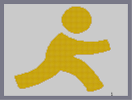Hover over the thumbnail for a full-size version.

Author littleshit author:littleshit n-art rated 2005-12-24 4 by 18 people. \$AIM IS BACK#littleshit#none#00000000000000000000000000000000000000000000000000000000000000000000000000000000000000000000000000000000000000000000000000000000000000000000000000000000000000000000000000000000000000000000000000000000000000000000000000000000000000000000000000000000000000000000000000000000000000000000000000000000000000000000000000000000000000000000000000000000000000000000000000000000000000000000000000000000000000000000000000000000000000000000000000000000000000000000000000000000000000000000000000000000000000000000000000000000000000000000000000000000000000000000000000000000000000000000000000000000000000000000000000000000000000000000000000000000000000000000000000000000000000000000000000000000000000000000000000000000000000000|5^672,564!0^90,456!0^90,462!0^90,468!0^96,468!0^96,474!0^102,480!0^96,480!0^96,486!0^102,486!0^102,492!0^102,498!0^114,504!0^108,498!0^108,504!0^108,510!0^108,516!0^114,516!0^114,522!0^120,522!0^120,528!0^126,528!0^138,528!0^144,528!0^150,528!0^132,528!0^174,522!0^174,522!0^168,522!0^168,522!0^162,522!0^156,522!0^150,522!0^186,516!0^180,516!0^174,516!0^204,510!0^198,510!0^186,510!0^192,516!0^192,510!0^210,510!0^228,504!0^222,504!0^222,504!0^216,504!0^210,504!0^210,504!0^240,498!0^240,498!0^228,498!0^222,498!0^234,498!0^252,492!0^252,492!0^246,492!0^240,492!0^228,492!0^234,492!0^264,486!0^258,486!0^252,486!0^246,492!0^276,480!0^270,480!0^264,480!0^282,474!0^276,474!0^294,468!0^282,468!0^282,468!0^288,468!0^306,462!0^300,462!0^300,462!0^294,462!0^312,456!0^312,456!0^306,456!0^318,450!0^312,450!0^312,450!0^288,474!0^300,468!0^312,462!0^318,456!0^324,450!0^330,450!0^336,444!0^330,444!0^324,444!0^342,438!0^336,438!0^336,438!0^348,432!0^348,432!0^342,432!0^354,426!0^348,426!0^348,426!0^384,420!0^378,420!0^372,420!0^366,414!0^360,420!0^366,420!0^372,420!0^378,426!0^384,426!0^354,420!0^402,426!0^390,426!0^396,426!0^402,432!0^402,432!0^408,432!0^414,432!0^414,432!0^426,438!0^426,438!0^420,438!0^438,444!0^438,444!0^432,444!0^432,438!0^426,438!0^420,432!0^408,426!0^402,420!0^396,420!0^414,426!0^438,432!0^390,420!0^450,450!0^444,444!0^456,444!0^456,450!0^462,456!0^450,444!0^444,450!0^456,456!0^462,462!0^468,462!0^474,462!0^480,462!0^468,468!0^480,468!0^474,468!0^474,474!0^486,474!0^486,474!0^480,474!0^480,480!0^486,480!0^492,480!0^486,486!0^492,486!0^498,486!0^498,492!0^492,492!0^504,492!0^498,498!0^510,498!0^510,498!0^504,498!0^504,504!0^510,504!0^510,504!0^516,504!0^516,504!0^510,510!0^522,510!0^522,510!0^522,510!0^504,510!0^510,516!0^516,516!0^522,516!0^522,516!0^522,516!0^516,510!0^516,522!0^522,522!0^522,522!0^528,522!0^528,522!0^522,528!0^534,528!0^528,528!0^528,534!0^522,534!0^534,534!0^534,534!0^522,540!0^528,540!0^540,540!0^540,540!0^534,546!0^528,540!0^534,540!0^528,546!0^546,540!0^552,546!0^552,546!0^546,546!0^540,546!0^564,540!0^558,540!0^552,540!0^552,540!0^576,540!0^570,540!0^558,546!0^594,534!0^594,534!0^588,534!0^582,534!0^576,528!0^570,534!0^600,528!0^594,528!0^582,528!0^600,528!0^606,522!0^606,522!0^594,522!0^588,522!0^576,534!0^606,528!0^588,528!0^612,522!0^600,522!0^612,516!0^606,510!0^612,498!0^612,504!0^612,504!0^612,510!0^606,492!0^606,498!0^600,486!0^600,486!0^600,492!0^600,498!0^600,498!0^594,480!0^594,492!0^594,492!0^594,474!0^588,468!0^594,480!0^594,486!0^594,492!0^606,504!0^588,474!0^588,480!0^588,462!0^582,468!0^582,462!0^582,456!0^582,456!0^576,456!0^576,450!0^576,456!0^576,468!0^576,468!0^576,450!0^576,456!0^576,462!0^570,444!0^570,450!0^570,456!0^570,462!0^564,438!0^564,432!0^564,450!0^564,456!0^564,444!0^558,432!0^558,426!0^558,438!0^558,444!0^558,444!0^552,420!0^552,432!0^552,426!0^546,420!0^540,414!0^534,408!0^546,414!0^540,408!0^528,402!0^534,402!0^528,396!0^522,396!0^522,390!0^516,390!0^552,432!0^546,426!0^546,426!0^540,420!0^534,414!0^534,414!0^528,408!0^522,402!0^516,396!0^618,510!0^618,516!0^516,384!0^510,384!0^516,396!0^516,390!0^510,390!0^510,378!0^504,378!0^504,384!0^510,384!0^510,384!0^498,378!0^504,372!0^498,372!0^492,372!0^492,372!0^492,366!0^486,366!0^480,366!0^486,360!0^480,360!0^474,360!0^468,360!0^474,354!0^468,354!0^462,354!0^456,354!0^456,354!0^456,348!0^450,348!0^444,342!0^444,348!0^438,342!0^438,330!0^438,330!0^438,324!0^438,330!0^438,336!0^444,324!0^438,318!0^438,318!0^444,318!0^450,318!0^456,318!0^462,312!0^516,318!0^462,318!0^468,318!0^468,318!0^480,312!0^510,318!0^504,318!0^498,318!0^486,318!0^480,318!0^468,318!0^492,318!0^474,318!0^486,324!0^492,324!0^504,324!0^534,324!0^528,324!0^522,324!0^516,324!0^516,324!0^510,324!0^504,324!0^498,324!0^540,324!0^522,318!0^474,312!0^468,312!0^456,312!0^444,318!0^450,312!0^450,312!0^432,324!0^432,330!0^432,336!0^432,336!0^438,312!0^444,312!0^528,312!0^522,312!0^522,312!0^516,312!0^510,312!0^498,312!0^486,312!0^486,312!0^492,312!0^492,306!0^504,318!0^498,312!0^504,312!0^528,318!0^528,318!0^534,318!0^546,312!0^570,318!0^570,318!0^564,318!0^558,318!0^546,318!0^540,318!0^546,312!0^480,324!0^474,324!0^468,324!0^558,324!0^552,324!0^546,324!0^588,312!0^588,318!0^582,318!0^576,318!0^552,318!0^582,312!0^576,312!0^576,312!0^570,312!0^558,312!0^552,312!0^552,312!0^540,306!0^540,312!0^534,312!0^564,312!0^594,312!0^600,312!0^606,312!0^606,306!0^612,312!0^618,312!0^630,306!0^624,306!0^618,306!0^612,306!0^600,306!0^588,306!0^582,306!0^594,306!0^636,306!0^642,300!0^636,300!0^636,300!0^630,300!0^624,300!0^648,300!0^648,300!0^654,300!0^654,294!0^654,282!0^654,282!0^654,288!0^660,288!0^660,294!0^642,294!0^642,300!0^642,294!0^648,294!0^630,294!0^636,294!0^648,288!0^648,288!0^648,282!0^648,282!0^648,276!0^648,270!0^648,264!0^648,258!0^654,276!0^654,270!0^654,264!0^648,252!0^648,258!0^642,252!0^636,252!0^636,246!0^642,246!0^642,240!0^630,240!0^630,240!0^630,240!0^624,240!0^624,240!0^636,240!0^624,246!0^618,246!0^630,246!0^624,252!0^624,252!0^618,252!0^612,252!0^612,252!0^606,252!0^600,252!0^594,252!0^588,252!0^582,252!0^612,246!0^606,246!0^594,246!0^600,246!0^576,252!0^576,252!0^570,252!0^564,252!0^558,252!0^552,252!0^552,252!0^546,252!0^540,252!0^534,252!0^528,252!0^528,252!0^528,252!0^528,252!0^522,252!0^522,252!0^516,252!0^510,252!0^510,252!0^504,252!0^504,252!0^504,252!0^498,252!0^492,252!0^486,246!0^498,246!0^492,246!0^480,246!0^480,246!0^474,246!0^468,246!0^462,246!0^456,246!0^450,246!0^486,252!0^486,252!0^474,252!0^468,252!0^462,252!0^456,252!0^480,252!0^600,258!0^594,258!0^588,258!0^576,258!0^564,258!0^546,264!0^492,258!0^510,264!0^516,258!0^522,258!0^534,258!0^546,258!0^564,258!0^570,258!0^570,258!0^558,258!0^552,258!0^540,258!0^534,258!0^516,258!0^504,258!0^582,258!0^456,240!0^450,240!0^444,240!0^438,240!0^444,234!0^438,234!0^432,234!0^426,234!0^426,234!0^420,234!0^426,228!0^420,228!0^414,228!0^408,228!0^432,240!0^444,246!0^408,222!0^408,222!0^402,222!0^396,222!0^390,228!0^396,216!0^402,216!0^390,216!0^390,216!0^384,216!0^390,216!0^396,222!0^390,210!0^384,210!0^378,210!0^372,210!0^378,204!0^372,204!0^372,204!0^366,204!0^366,204!0^360,204!0^372,198!0^366,198!0^354,210!0^348,210!0^360,210!0^354,204!0^528,264!0^558,264!0^558,270!0^558,276!0^564,288!0^570,264!0^570,264!0^582,288!0^606,270!0^612,270!0^594,264!0^642,258!0^642,258!0^618,264!0^630,258!0^630,258!0^630,264!0^348,216!0^342,222!0^342,216!0^480,42!0^480,42!0^474,42!0^474,42!0^468,42!0^462,42!0^462,42!0^456,42!0^450,42!0^450,42!0^444,42!0^438,42!0^438,48!0^438,48!0^432,48!0^426,48!0^426,48!0^432,42!0^414,54!0^420,54!0^420,54!0^426,54!0^420,48!0^432,54!0^402,60!0^408,60!0^414,60!0^414,60!0^414,60!0^414,60!0^420,60!0^420,60!0^396,66!0^402,66!0^408,66!0^408,66!0^408,66!0^414,66!0^390,72!0^396,72!0^402,72!0^408,72!0^414,72!0^420,66!0^390,78!0^396,78!0^402,78!0^408,78!0^408,78!0^384,84!0^390,84!0^396,84!0^402,84!0^384,90!0^396,96!0^402,90!0^402,90!0^390,90!0^390,90!0^378,90!0^402,90!0^378,96!0^378,102!0^378,102!0^378,108!0^378,114!0^378,126!0^378,138!0^378,138!0^378,132!0^378,126!0^384,120!0^372,102!0^372,108!0^372,120!0^372,126!0^372,114!0^378,150!0^378,144!0^384,156!0^384,144!0^384,144!0^384,138!0^384,132!0^384,120!0^378,114!0^378,120!0^384,132!0^384,126!0^384,150!0^384,96!0^384,102!0^384,114!0^384,120!0^384,108!0^396,90!0^372,132!0^390,162!0^390,156!0^396,168!0^396,162!0^402,174!0^402,168!0^408,174!0^408,180!0^492,48!0^486,48!0^480,48!0^498,48!0^504,54!0^498,54!0^498,54!0^492,54!0^510,60!0^510,60!0^498,60!0^498,60!0^510,54!0^516,60!0^498,60!0^504,60!0^522,66!0^516,66!0^510,66!0^510,66!0^504,66!0^528,72!0^522,72!0^522,72!0^522,72!0^516,72!0^510,72!0^534,78!0^528,78!0^522,78!0^516,78!0^516,78!0^534,84!0^528,84!0^522,84!0^516,84!0^540,90!0^540,90!0^534,90!0^528,90!0^528,90!0^540,96!0^540,96!0^534,96!0^540,102!0^540,108!0^540,108!0^540,120!0^540,120!0^540,126!0^540,114!0^546,108!0^546,108!0^546,114!0^546,120!0^546,126!0^546,132!0^540,132!0^540,138!0^540,138!0^534,144!0^534,150!0^534,144!0^540,144!0^528,156!0^534,138!0^534,138!0^534,132!0^528,150!0^534,156!0^540,150!0^528,162!0^528,168!0^522,168!0^522,162!0^534,102!0^534,108!0^534,120!0^534,126!0^534,114!0^522,174!0^516,174!0^516,168!0^516,180!0^510,180!0^510,174!0^510,174!0^510,174!0^504,186!0^504,180!0^504,180!0^504,180!0^498,186!0^498,174!0^498,174!0^498,180!0^498,180!0^492,192!0^492,186!0^492,186!0^492,180!0^492,180!0^486,192!0^480,192!0^486,180!0^486,180!0^486,186!0^486,186!0^474,198!0^468,192!0^468,192!0^468,198!0^462,198!0^456,198!0^450,198!0^444,198!0^480,192!0^474,192!0^468,192!0^462,192!0^450,192!0^444,192!0^444,192!0^426,192!0^438,192!0^456,192!0^432,192!0^480,186!0^474,186!0^474,186!0^462,186!0^462,186!0^450,186!0^444,186!0^432,186!0^426,186!0^414,186!0^414,180!0^420,180!0^420,186!0^426,186!0^438,186!0^450,186!0^468,186!0^462,186!0^546,102!0^546,138!0^474,48!0^468,54!0^462,54!0^444,48!0^450,48!0^456,42!0^468,48!0^474,48!0^462,48!0^462,48!0^456,48!0^456,48!0^336,228!0^336,222!0^336,228!0^330,228!0^336,222!0^324,234!0^330,234!0^318,240!0^330,240!0^312,246!0^312,246!0^312,246!0^318,246!0^324,246!0^324,240!0^324,252!0^318,252!0^312,252!0^306,252!0^306,258!0^306,258!0^312,258!0^318,258!0^318,258!0^300,264!0^306,264!0^312,264!0^318,264!0^318,258!0^534,162!0^300,270!0^300,276!0^306,276!0^306,276!0^306,270!0^318,270!0^318,276!0^300,276!0^294,276!0^294,282!0^288,282!0^288,288!0^294,282!0^306,276!0^312,270!0^312,276!0^312,282!0^312,282!0^306,282!0^306,282!0^300,282!0^294,288!0^306,288!0^312,288!0^318,288!0^288,294!0^288,294!0^288,300!0^288,300!0^300,288!0^300,294!0^294,300!0^294,306!0^294,294!0^300,294!0^282,300!0^288,306!0^282,306!0^282,318!0^282,318!0^288,312!0^288,312!0^288,318!0^288,324!0^282,312!0^276,318!0^276,318!0^276,324!0^276,336!0^282,324!0^282,324!0^282,336!0^282,336!0^276,330!0^282,330!0^276,348!0^276,342!0^276,348!0^276,354!0^270,354!0^270,360!0^270,360!0^270,366!0^264,366!0^264,372!0^270,372!0^276,372!0^276,366!0^282,354!0^288,348!0^282,342!0^288,348!0^270,366!0^276,360!0^282,348!0^264,378!0^258,378!0^252,378!0^264,372!0^270,384!0^246,384!0^252,384!0^258,384!0^258,384!0^264,384!0^276,378!0^258,372!0^240,390!0^246,390!0^252,384!0^252,384!0^252,390!0^252,390!0^234,396!0^240,396!0^240,396!0^228,402!0^228,402!0^234,402!0^240,402!0^228,408!0^222,408!0^240,408!0^234,408!0^246,396!0^228,408!0^228,414!0^222,414!0^222,414!0^216,414!0^210,414!0^216,420!0^210,420!0^210,420!0^198,420!0^204,420!0^216,420!0^210,426!0^204,426!0^204,426!0^198,426!0^192,426!0^186,426!0^198,432!0^198,432!0^192,432!0^180,432!0^180,432!0^174,432!0^186,432!0^156,438!0^162,438!0^168,438!0^174,438!0^180,438!0^180,438!0^168,444!0^162,444!0^156,444!0^150,438!0^150,444!0^150,444!0^144,444!0^144,444!0^138,444!0^132,444!0^150,450!0^144,450!0^138,450!0^132,450!0^126,450!0^126,450!0^120,450!0^108,450!0^114,450!0^120,456!0^120,456!0^114,456!0^114,456!0^108,456!0^96,456!0^96,456!0^102,456!0^102,456!0^450,90!0^444,102!0^450,108!0^450,84!0^444,54!0^438,48!0^432,60!0^420,90!0^432,108!0^432,66!0^438,78!0^426,108!0^432,90!0^438,90!0^438,108!0^438,96!0^444,78!0^426,90!0^426,168!0^450,150!0^468,132!0^444,150!0^462,114!0^390,96!0^390,96!0^390,102!0^390,108!0^390,120!0^390,126!0^390,138!0^396,144!0^390,138!0^390,126!0^390,108!0^390,114!0^390,120!0^396,120!0^390,132!0^390,144!0^390,150!0^396,156!0^396,156!0^396,150!0^402,150!0^402,144!0^402,138!0^396,138!0^396,126!0^396,120!0^396,114!0^396,102!0^396,102!0^396,108!0^396,114!0^396,132!0^402,96!0^396,102!0^402,102!0^402,102!0^396,108!0^402,108!0^402,108!0^402,120!0^402,126!0^402,132!0^402,150!0^402,156!0^402,162!0^402,114!0^402,120!0^408,84!0^408,84!0^408,102!0^408,108!0^408,126!0^408,138!0^408,156!0^402,162!0^402,162!0^408,168!0^408,162!0^414,150!0^414,144!0^408,144!0^408,150!0^408,132!0^408,120!0^408,114!0^408,90!0^408,96!0^414,78!0^414,84!0^414,96!0^414,102!0^414,114!0^414,126!0^414,138!0^414,120!0^414,90!0^414,108!0^414,132!0^474,90!0^468,108!0^468,120!0^474,126!0^450,84!0^426,78!0^438,108!0^438,108!0^432,132!0^432,126!0^432,126!0^426,138!0^438,96!0^420,84!0^426,138!0^450,126!0^474,102!0^468,138!0^492,108!0^498,120!0^480,138!0^546,108!0^420,78!0^420,72!0^426,72!0^426,60!0^426,66!0^432,72!0^432,72!0^432,84!0^432,84!0^426,84!0^432,84!0^438,84!0^444,84!0^444,84!0^444,90!0^444,90!0^432,78!0^438,54!0^438,60!0^438,66!0^438,66!0^438,72!0^444,72!0^444,72!0^450,66!0^444,66!0^444,60!0^450,54!0^450,60!0^450,66!0^450,72!0^450,78!0^456,54!0^456,54!0^456,60!0^456,72!0^456,78!0^456,78!0^456,96!0^456,66!0^456,78!0^456,84!0^456,90!0^456,90!0^468,60!0^462,60!0^462,60!0^462,66!0^462,78!0^462,84!0^462,96!0^462,90!0^462,72!0^468,66!0^468,66!0^468,72!0^468,78!0^468,84!0^468,90!0^468,96!0^474,60!0^474,60!0^474,72!0^474,78!0^474,84!0^474,90!0^474,66!0^474,60!0^474,54!0^480,54!0^480,54!0^486,54!0^480,60!0^480,66!0^480,72!0^480,84!0^480,84!0^480,90!0^480,78!0^486,60!0^492,60!0^498,66!0^492,66!0^492,66!0^486,66!0^486,78!0^486,72!0^486,72!0^492,72!0^498,72!0^504,72!0^510,78!0^504,78!0^504,78!0^498,78!0^486,84!0^492,84!0^492,84!0^504,84!0^504,84!0^498,84!0^492,78!0^510,84!0^486,90!0^498,90!0^498,90!0^498,90!0^510,84!0^516,90!0^522,90!0^510,96!0^510,90!0^504,90!0^492,90!0^528,96!0^528,96!0^528,114!0^528,120!0^528,126!0^528,138!0^528,132!0^528,144!0^528,102!0^528,102!0^528,108!0^522,96!0^516,96!0^516,96!0^522,102!0^522,102!0^522,108!0^522,114!0^522,114!0^522,120!0^522,126!0^522,132!0^522,144!0^522,144!0^522,150!0^522,156!0^522,138!0^516,102!0^510,102!0^504,96!0^498,96!0^492,96!0^486,96!0^474,96!0^480,96!0^480,102!0^486,102!0^492,102!0^498,102!0^504,108!0^504,102!0^498,108!0^498,108!0^504,108!0^510,108!0^516,108!0^516,120!0^516,132!0^516,144!0^516,156!0^516,156!0^516,150!0^516,138!0^516,126!0^516,114!0^510,114!0^504,114!0^504,114!0^492,114!0^510,120!0^510,120!0^510,126!0^504,132!0^498,108!0^498,114!0^516,162!0^504,174!0^510,168!0^510,156!0^510,138!0^510,132!0^510,138!0^510,150!0^510,162!0^510,168!0^510,144!0^504,126!0^504,126!0^504,120!0^498,126!0^498,138!0^498,144!0^498,150!0^498,168!0^498,168!0^498,162!0^498,156!0^498,132!0^504,144!0^504,150!0^504,156!0^504,168!0^504,138!0^504,156!0^486,114!0^492,120!0^492,126!0^492,132!0^492,138!0^492,150!0^492,156!0^492,168!0^492,168!0^492,174!0^492,162!0^504,168!0^504,162!0^486,108!0^480,108!0^480,108!0^474,114!0^468,114!0^480,114!0^480,120!0^486,126!0^474,120!0^474,102!0^474,108!0^480,120!0^486,132!0^486,120!0^480,126!0^480,138!0^486,138!0^474,132!0^474,132!0^474,138!0^492,144!0^486,144!0^480,144!0^480,144!0^468,144!0^480,132!0^486,150!0^486,162!0^486,168!0^486,174!0^486,168!0^486,162!0^480,156!0^474,102!0^468,102!0^462,102!0^462,102!0^450,102!0^450,102!0^450,102!0^444,102!0^432,102!0^432,102!0^450,90!0^450,96!0^450,96!0^438,96!0^420,102!0^426,96!0^426,96!0^426,108!0^420,96!0^432,96!0^426,102!0^444,96!0^438,102!0^456,96!0^456,102!0^456,108!0^462,108!0^462,108!0^444,108!0^420,108!0^420,108!0^420,120!0^420,120!0^420,132!0^420,138!0^414,144!0^414,150!0^456,114!0^450,114!0^450,114!0^444,108!0^438,108!0^438,114!0^438,114!0^426,114!0^420,114!0^444,114!0^432,114!0^426,132!0^426,126!0^426,120!0^420,126!0^462,120!0^462,120!0^456,120!0^456,120!0^450,120!0^444,120!0^438,120!0^432,120!0^468,126!0^462,126!0^462,126!0^456,126!0^450,126!0^444,126!0^438,126!0^438,132!0^444,132!0^450,132!0^456,132!0^456,132!0^462,132!0^462,138!0^462,138!0^456,138!0^456,138!0^450,138!0^444,138!0^438,138!0^420,150!0^432,144!0^438,144!0^444,144!0^450,144!0^462,144!0^468,144!0^468,144!0^456,144!0^432,138!0^426,144!0^420,144!0^474,144!0^474,150!0^474,150!0^480,150!0^468,150!0^462,150!0^456,150!0^438,150!0^432,150!0^426,150!0^414,156!0^426,156!0^426,156!0^420,156!0^432,156!0^438,156!0^450,156!0^462,156!0^468,156!0^474,156!0^480,156!0^486,156!0^456,156!0^444,156!0^480,162!0^480,162!0^468,162!0^468,162!0^462,162!0^456,162!0^474,162!0^444,162!0^438,156!0^432,156!0^432,162!0^426,162!0^420,162!0^414,162!0^438,162!0^450,162!0^414,168!0^414,174!0^420,174!0^420,168!0^480,168!0^474,168!0^474,168!0^468,168!0^462,168!0^462,168!0^456,168!0^456,168!0^450,168!0^432,162!0^432,168!0^438,168!0^444,168!0^444,168!0^480,174!0^474,174!0^468,174!0^468,174!0^462,174!0^456,168!0^450,168!0^456,174!0^450,174!0^450,174!0^444,174!0^444,174!0^438,174!0^432,174!0^426,168!0^426,174!0^432,180!0^432,180!0^438,180!0^444,180!0^456,180!0^462,180!0^480,180!0^474,180!0^468,180!0^468,180!0^450,180!0^426,180!0^456,186!0^636,252!0^636,252!0^642,264!0^642,264!0^642,270!0^642,270!0^642,276!0^642,276!0^642,288!0^642,288!0^642,288!0^630,288!0^642,276!0^624,252!0^630,246!0^636,258!0^630,252!0^636,270!0^636,264!0^624,264!0^624,252!0^624,258!0^618,258!0^618,258!0^612,258!0^642,282!0^630,270!0^636,270!0^636,276!0^636,282!0^636,288!0^630,276!0^630,276!0^624,270!0^624,270!0^624,282!0^624,288!0^618,294!0^630,282!0^624,276!0^624,294!0^618,300!0^618,294!0^618,288!0^618,288!0^618,282!0^618,276!0^618,270!0^612,264!0^612,264!0^612,270!0^612,270!0^606,276!0^606,282!0^612,294!0^612,300!0^612,300!0^612,288!0^612,276!0^612,270!0^612,282!0^612,288!0^612,264!0^606,264!0^606,264!0^606,270!0^594,270!0^606,258!0^528,258!0^528,258!0^504,258!0^510,258!0^600,264!0^600,264!0^600,276!0^594,282!0^594,294!0^600,300!0^600,300!0^600,294!0^600,270!0^600,276!0^606,288!0^606,294!0^606,294!0^606,300!0^600,282!0^600,288!0^600,288!0^600,288!0^594,288!0^588,288!0^582,282!0^594,276!0^594,276!0^582,276!0^576,276!0^576,276!0^576,264!0^576,264!0^588,264!0^588,264!0^588,276!0^588,282!0^594,270!0^588,270!0^582,270!0^576,270!0^582,264!0^594,300!0^594,300!0^588,300!0^558,300!0^570,300!0^570,300!0^576,300!0^576,300!0^564,300!0^582,300!0^582,294!0^588,294!0^582,300!0^576,300!0^564,294!0^558,294!0^582,294!0^576,294!0^570,288!0^570,288!0^576,282!0^576,288!0^570,294!0^570,282!0^570,276!0^570,276!0^564,264!0^564,270!0^564,276!0^564,288!0^564,288!0^570,270!0^564,288!0^558,288!0^564,282!0^564,282!0^558,288!0^576,306!0^570,306!0^570,306!0^558,306!0^546,306!0^552,306!0^564,306!0^546,300!0^552,300!0^552,300!0^552,294!0^552,288!0^552,288!0^558,282!0^558,282!0^552,282!0^552,276!0^552,270!0^552,264!0^540,270!0^546,270!0^546,276!0^546,282!0^546,288!0^546,294!0^540,300!0^540,288!0^540,270!0^540,264!0^534,264!0^540,282!0^540,288!0^540,294!0^540,276!0^534,264!0^534,270!0^534,276!0^534,282!0^534,282!0^534,288!0^534,294!0^534,294!0^534,300!0^528,306!0^534,306!0^528,300!0^528,294!0^528,294!0^528,288!0^528,282!0^528,276!0^528,270!0^522,264!0^522,264!0^522,270!0^522,276!0^522,282!0^522,288!0^522,294!0^522,306!0^522,306!0^522,300!0^516,264!0^516,264!0^516,264!0^516,276!0^516,282!0^516,282!0^516,288!0^516,300!0^516,306!0^516,306!0^516,300!0^516,294!0^516,282!0^516,270!0^504,270!0^504,276!0^510,270!0^510,270!0^510,276!0^510,282!0^510,288!0^510,294!0^504,300!0^510,300!0^504,306!0^504,264!0^498,258!0^492,264!0^486,264!0^492,264!0^492,264!0^498,270!0^504,276!0^510,288!0^510,300!0^510,300!0^504,264!0^492,264!0^492,264!0^498,264!0^498,264!0^504,276!0^504,282!0^504,288!0^510,294!0^516,306!0^510,306!0^498,306!0^498,300!0^492,288!0^492,276!0^498,270!0^498,276!0^498,276!0^498,282!0^498,288!0^498,294!0^504,294!0^492,264!0^492,264!0^492,264!0^492,270!0^492,282!0^492,300!0^492,294!0^486,258!0^486,258!0^486,264!0^486,270!0^486,264!0^486,270!0^486,270!0^486,282!0^486,282!0^486,288!0^486,294!0^486,300!0^486,306!0^486,276!0^480,258!0^474,264!0^480,252!0^480,264!0^486,270!0^486,288!0^480,306!0^480,300!0^480,300!0^480,294!0^480,282!0^474,276!0^480,270!0^480,276!0^480,288!0^474,258!0^474,264!0^474,276!0^474,276!0^474,288!0^474,300!0^474,300!0^474,306!0^468,294!0^468,288!0^474,288!0^468,276!0^474,270!0^474,264!0^474,294!0^474,282!0^468,258!0^468,258!0^468,264!0^468,264!0^468,270!0^468,282!0^468,288!0^468,306!0^468,306!0^468,300!0^462,258!0^462,258!0^462,270!0^462,276!0^462,282!0^462,300!0^462,306!0^462,312!0^462,300!0^462,294!0^468,288!0^468,282!0^462,282!0^462,264!0^462,288!0^456,258!0^456,264!0^456,270!0^456,270!0^456,276!0^456,288!0^450,294!0^456,306!0^462,306!0^462,300!0^456,282!0^456,288!0^462,300!0^456,300!0^456,294!0^450,306!0^450,306!0^450,294!0^450,288!0^450,276!0^456,264!0^450,252!0^450,258!0^450,264!0^450,270!0^450,282!0^450,282!0^450,294!0^450,300!0^444,306!0^444,306!0^444,294!0^444,294!0^444,282!0^444,282!0^444,270!0^444,252!0^444,258!0^444,264!0^444,270!0^444,276!0^444,282!0^444,288!0^444,300!0^444,300!0^438,306!0^438,306!0^438,300!0^438,246!0^438,252!0^438,258!0^438,264!0^438,270!0^438,282!0^438,288!0^438,294!0^438,282!0^432,318!0^432,318!0^432,306!0^432,306!0^432,294!0^438,282!0^432,252!0^432,252!0^432,258!0^432,270!0^432,276!0^432,282!0^432,294!0^438,276!0^432,252!0^432,246!0^432,264!0^432,288!0^432,300!0^432,312!0^366,210!0^354,216!0^354,216!0^354,216!0^360,216!0^360,216!0^366,216!0^366,216!0^372,216!0^378,216!0^390,222!0^390,222!0^384,222!0^378,222!0^372,222!0^366,222!0^354,222!0^354,222!0^354,216!0^360,222!0^348,222!0^342,228!0^348,228!0^354,228!0^354,228!0^360,228!0^366,228!0^372,228!0^384,228!0^390,228!0^396,228!0^396,228!0^378,228!0^402,228!0^414,234!0^414,234!0^408,234!0^396,234!0^396,234!0^384,234!0^378,234!0^366,234!0^360,234!0^354,234!0^348,234!0^336,234!0^354,234!0^366,234!0^372,234!0^396,234!0^396,240!0^390,234!0^402,234!0^342,234!0^426,240!0^426,240!0^420,240!0^408,240!0^408,240!0^402,240!0^408,240!0^414,240!0^390,240!0^384,240!0^378,240!0^372,246!0^360,246!0^342,240!0^348,240!0^354,240!0^360,240!0^366,240!0^372,240!0^336,240!0^426,246!0^426,246!0^420,246!0^414,246!0^414,246!0^408,252!0^402,252!0^408,240!0^402,240!0^402,246!0^390,246!0^384,246!0^366,246!0^366,246!0^384,252!0^396,246!0^402,246!0^408,246!0^402,246!0^378,246!0^372,246!0^366,246!0^354,246!0^348,246!0^336,246!0^330,246!0^336,252!0^342,240!0^348,246!0^342,246!0^342,252!0^330,252!0^426,252!0^420,252!0^414,252!0^396,252!0^390,252!0^378,252!0^378,252!0^366,252!0^360,252!0^342,252!0^348,246!0^348,252!0^348,252!0^354,252!0^354,252!0^366,252!0^372,252!0^426,258!0^420,258!0^420,258!0^414,258!0^414,258!0^408,258!0^408,258!0^402,258!0^396,258!0^390,258!0^390,258!0^384,258!0^372,258!0^366,258!0^378,258!0^372,258!0^360,258!0^354,252!0^336,252!0^324,258!0^330,258!0^336,258!0^342,258!0^354,258!0^354,258!0^354,258!0^348,258!0^324,264!0^324,264!0^336,264!0^426,336!0^426,336!0^420,336!0^414,336!0^408,336!0^396,336!0^390,336!0^384,336!0^402,336!0^378,342!0^378,336!0^378,336!0^372,336!0^366,336!0^360,336!0^348,336!0^342,336!0^288,336!0^294,336!0^300,336!0^312,336!0^318,336!0^324,336!0^336,336!0^330,336!0^330,336!0^306,336!0^354,336!0^426,264!0^426,270!0^426,282!0^420,288!0^420,294!0^420,318!0^426,330!0^420,318!0^420,282!0^366,276!0^390,312!0^414,294!0^402,288!0^342,312!0^330,312!0^354,282!0^378,270!0^384,288!0^414,294!0^408,270!0^402,282!0^360,306!0^348,300!0^372,282!0^378,306!0^378,300!0^372,276!0^372,276!0^354,282!0^354,282!0^330,300!0^330,306!0^384,282!0^384,282!0^378,282!0^366,306!0^384,288!0^408,282!0^402,282!0^396,270!0^396,282!0^372,276!0^396,276!0^366,282!0^324,276!0^354,294!0^378,294!0^378,306!0^414,288!0^402,306!0^420,318!0^420,294!0^414,300!0^426,318!0^426,300!0^426,288!0^426,270!0^426,282!0^426,288!0^426,300!0^426,312!0^426,324!0^426,324!0^432,306!0^426,300!0^426,276!0^426,294!0^426,294!0^426,306!0^420,264!0^420,270!0^420,270!0^420,282!0^420,276!0^420,300!0^420,306!0^420,312!0^420,324!0^420,330!0^414,264!0^414,270!0^414,270!0^414,276!0^408,282!0^408,288!0^414,330!0^414,324!0^414,318!0^414,312!0^414,306!0^414,288!0^408,264!0^414,282!0^408,276!0^402,276!0^402,264!0^402,270!0^408,330!0^408,324!0^408,324!0^408,318!0^408,312!0^408,300!0^408,300!0^414,294!0^408,306!0^408,294!0^402,294!0^402,300!0^402,306!0^402,312!0^402,318!0^402,324!0^402,330!0^396,330!0^402,318!0^396,288!0^396,294!0^396,300!0^396,300!0^396,306!0^390,318!0^390,324!0^396,324!0^396,318!0^396,318!0^396,312!0^390,330!0^390,306!0^390,300!0^390,294!0^390,288!0^390,282!0^390,276!0^390,264!0^396,264!0^396,264!0^384,270!0^384,270!0^378,264!0^366,264!0^384,264!0^390,270!0^384,276!0^378,276!0^384,294!0^384,294!0^384,300!0^384,300!0^384,312!0^384,318!0^384,330!0^384,330!0^390,306!0^384,306!0^384,324!0^378,330!0^378,324!0^378,324!0^378,318!0^378,312!0^378,306!0^378,288!0^372,288!0^366,288!0^366,288!0^372,264!0^372,270!0^366,270!0^366,264!0^360,264!0^354,264!0^354,264!0^342,264!0^324,264!0^330,264!0^342,270!0^348,264!0^324,270!0^324,270!0^330,270!0^336,270!0^336,270!0^342,270!0^348,270!0^354,270!0^360,270!0^360,270!0^360,282!0^360,276!0^360,276!0^360,276!0^354,276!0^348,276!0^342,276!0^336,270!0^330,276!0^318,282!0^330,282!0^336,282!0^342,282!0^348,282!0^348,282!0^336,282!0^324,282!0^336,276!0^372,330!0^372,330!0^366,330!0^360,330!0^354,330!0^342,330!0^336,330!0^330,330!0^324,330!0^318,330!0^306,324!0^300,324!0^288,330!0^300,330!0^306,330!0^312,330!0^294,330!0^354,330!0^348,336!0^348,330!0^372,294!0^372,294!0^372,306!0^372,306!0^372,312!0^366,324!0^372,318!0^372,318!0^372,312!0^372,300!0^372,324!0^366,318!0^366,312!0^366,306!0^360,300!0^366,294!0^366,300!0^366,300!0^360,300!0^360,288!0^360,288!0^354,288!0^348,288!0^336,288!0^330,288!0^324,288!0^342,288!0^360,294!0^360,324!0^360,318!0^360,312!0^354,300!0^354,300!0^354,306!0^354,312!0^354,318!0^354,318!0^354,324!0^348,318!0^348,318!0^348,306!0^348,306!0^348,318!0^348,330!0^348,318!0^348,312!0^348,324!0^342,294!0^342,294!0^330,294!0^324,294!0^312,294!0^306,294!0^312,294!0^318,294!0^330,294!0^342,300!0^348,300!0^348,294!0^336,288!0^336,294!0^336,306!0^336,312!0^336,318!0^336,318!0^330,318!0^336,324!0^336,318!0^342,318!0^342,324!0^342,306!0^342,306!0^342,300!0^330,330!0^330,324!0^336,300!0^324,324!0^324,318!0^324,312!0^324,306!0^330,300!0^324,300!0^324,300!0^324,300!0^318,300!0^318,300!0^312,300!0^306,300!0^300,300!0^300,312!0^294,324!0^294,318!0^294,312!0^300,306!0^306,306!0^312,306!0^318,306!0^318,306!0^318,312!0^318,318!0^318,318!0^318,318!0^312,324!0^312,312!0^306,312!0^306,312!0^306,318!0^300,318!0^312,318!0^318,324!0^564,534!0^564,534!0^564,534!0^552,540!0^552,540!0^540,534!0^552,534!0^552,534!0^558,534!0^552,534!0^546,534!0^606,516!0^606,516!0^606,522!0^576,528!0^576,528!0^564,528!0^558,528!0^546,528!0^540,528!0^600,504!0^600,504!0^600,510!0^594,516!0^588,516!0^588,504!0^594,498!0^594,504!0^594,510!0^588,510!0^588,480!0^588,492!0^588,498!0^588,498!0^588,486!0^600,516!0^582,474!0^582,474!0^582,480!0^582,486!0^582,498!0^582,504!0^582,516!0^582,522!0^582,516!0^582,510!0^582,510!0^582,492!0^576,474!0^576,480!0^576,480!0^576,492!0^576,492!0^576,498!0^576,510!0^576,522!0^576,528!0^576,516!0^576,516!0^576,504!0^576,504!0^576,486!0^570,468!0^570,468!0^570,480!0^570,486!0^570,498!0^570,510!0^570,522!0^570,528!0^564,522!0^564,516!0^570,516!0^570,516!0^570,504!0^576,504!0^576,492!0^564,474!0^570,474!0^570,492!0^564,456!0^564,462!0^564,462!0^564,480!0^564,480!0^570,498!0^570,504!0^564,510!0^564,504!0^558,504!0^558,498!0^558,492!0^564,480!0^564,486!0^564,492!0^564,492!0^564,492!0^564,492!0^564,468!0^564,498!0^558,450!0^558,450!0^558,456!0^558,456!0^558,468!0^558,480!0^558,486!0^558,492!0^558,480!0^558,474!0^558,462!0^552,510!0^558,510!0^558,510!0^558,516!0^558,522!0^552,528!0^552,516!0^552,516!0^552,510!0^552,522!0^552,438!0^552,438!0^552,450!0^552,462!0^552,468!0^552,486!0^552,498!0^552,504!0^552,498!0^552,492!0^552,486!0^552,480!0^552,474!0^552,456!0^552,444!0^546,432!0^546,438!0^546,450!0^546,456!0^546,474!0^546,444!0^546,462!0^546,468!0^546,480!0^546,486!0^546,498!0^546,498!0^546,510!0^546,522!0^546,528!0^540,516!0^540,510!0^546,492!0^546,504!0^546,516!0^540,426!0^540,426!0^540,438!0^540,444!0^540,450!0^540,456!0^540,462!0^540,474!0^540,486!0^540,498!0^540,528!0^540,522!0^534,522!0^540,504!0^540,492!0^540,486!0^540,474!0^540,468!0^540,480!0^540,432!0^534,420!0^534,420!0^534,432!0^534,432!0^534,438!0^528,450!0^534,426!0^534,438!0^534,444!0^534,450!0^534,456!0^534,456!0^534,462!0^534,468!0^534,474!0^534,480!0^534,492!0^534,498!0^534,510!0^534,510!0^522,516!0^528,510!0^534,510!0^534,510!0^534,516!0^522,516!0^528,516!0^534,504!0^534,486!0^528,420!0^528,420!0^528,426!0^528,426!0^528,432!0^528,438!0^528,438!0^528,444!0^528,450!0^528,414!0^528,456!0^528,456!0^528,468!0^528,468!0^528,480!0^528,486!0^528,492!0^528,492!0^528,504!0^528,474!0^528,462!0^528,498!0^522,504!0^522,498!0^522,498!0^522,492!0^522,486!0^522,474!0^522,468!0^522,456!0^522,408!0^522,408!0^522,408!0^522,414!0^522,414!0^522,420!0^522,420!0^522,426!0^522,438!0^522,438!0^522,444!0^522,450!0^522,432!0^522,462!0^522,480!0^516,402!0^516,402!0^516,408!0^516,414!0^516,414!0^516,420!0^516,426!0^510,432!0^516,432!0^516,432!0^516,438!0^516,444!0^516,444!0^516,450!0^516,456!0^516,462!0^516,468!0^516,480!0^516,480!0^516,486!0^510,504!0^516,498!0^516,498!0^516,486!0^516,486!0^522,474!0^516,474!0^516,492!0^516,396!0^510,396!0^510,396!0^510,402!0^510,408!0^510,408!0^510,414!0^510,420!0^510,426!0^510,432!0^510,438!0^510,450!0^510,450!0^510,462!0^510,468!0^510,474!0^510,480!0^510,486!0^510,492!0^510,492!0^510,444!0^510,456!0^504,390!0^498,396!0^498,402!0^504,396!0^504,396!0^504,402!0^504,414!0^504,426!0^504,432!0^498,450!0^498,456!0^504,486!0^504,474!0^504,474!0^504,468!0^504,468!0^504,444!0^504,438!0^504,438!0^504,450!0^504,450!0^504,456!0^504,474!0^504,480!0^504,462!0^504,408!0^504,420!0^498,384!0^498,384!0^492,396!0^498,390!0^498,414!0^498,414!0^498,408!0^498,414!0^498,420!0^498,426!0^498,426!0^498,432!0^498,438!0^498,438!0^498,444!0^498,444!0^498,450!0^498,456!0^498,456!0^498,468!0^498,468!0^498,474!0^498,456!0^498,456!0^498,462!0^498,480!0^492,378!0^492,378!0^486,384!0^486,390!0^408,420!0^408,420!0^408,420!0^414,414!0^420,414!0^372,390!0^372,390!0^378,390!0^384,390!0^390,390!0^402,390!0^414,390!0^420,390!0^450,396!0^492,402!0^492,402!0^474,402!0^432,402!0^426,408!0^462,408!0^480,408!0^450,408!0^444,408!0^456,408!0^468,408!0^480,408!0^486,408!0^486,408!0^474,408!0^438,408!0^426,408!0^498,408!0^492,408!0^492,408!0^492,420!0^492,426!0^492,432!0^492,438!0^492,450!0^492,456!0^492,468!0^492,474!0^492,462!0^492,450!0^492,444!0^492,408!0^486,414!0^486,414!0^486,426!0^486,432!0^486,438!0^486,444!0^486,456!0^486,462!0^486,462!0^486,444!0^486,468!0^486,450!0^486,420!0^492,414!0^480,414!0^480,414!0^480,420!0^480,426!0^480,432!0^480,438!0^480,444!0^480,450!0^480,456!0^474,456!0^474,438!0^474,438!0^474,426!0^474,414!0^474,408!0^474,414!0^474,414!0^474,420!0^474,426!0^474,432!0^474,438!0^474,444!0^480,450!0^474,450!0^468,456!0^468,456!0^468,450!0^468,450!0^468,444!0^468,444!0^468,432!0^468,426!0^468,414!0^468,414!0^468,420!0^468,426!0^468,438!0^468,438!0^462,450!0^462,450!0^462,450!0^462,444!0^462,438!0^462,438!0^456,420!0^462,414!0^462,414!0^462,426!0^462,426!0^462,432!0^462,420!0^456,438!0^456,432!0^456,432!0^456,426!0^456,420!0^456,414!0^450,414!0^450,420!0^450,420!0^450,426!0^450,432!0^450,438!0^438,438!0^438,438!0^444,438!0^444,432!0^444,426!0^444,420!0^444,420!0^438,414!0^438,414!0^432,414!0^432,414!0^432,408!0^444,414!0^438,426!0^438,426!0^426,432!0^432,426!0^432,420!0^438,420!0^432,420!0^426,420!0^426,420!0^420,420!0^414,420!0^426,414!0^420,426!0^426,426!0^432,432!0^492,390!0^492,384!0^486,372!0^486,372!0^486,378!0^486,384!0^486,390!0^486,396!0^486,396!0^480,402!0^480,390!0^480,384!0^474,378!0^480,372!0^480,384!0^480,390!0^486,396!0^486,396!0^474,396!0^474,390!0^474,384!0^480,378!0^486,402!0^480,396!0^474,366!0^474,366!0^474,372!0^468,402!0^468,396!0^468,396!0^468,390!0^468,384!0^468,378!0^468,372!0^468,372!0^468,366!0^462,360!0^462,360!0^462,366!0^462,372!0^462,372!0^462,378!0^462,384!0^462,390!0^462,396!0^462,402!0^456,360!0^456,360!0^456,366!0^456,372!0^456,378!0^456,378!0^456,384!0^456,390!0^456,396!0^456,402!0^456,402!0^450,354!0^450,354!0^450,360!0^450,366!0^450,366!0^450,378!0^450,384!0^450,390!0^450,396!0^450,402!0^450,372!0^444,354!0^444,354!0^444,360!0^444,366!0^444,372!0^450,378!0^450,378!0^450,384!0^450,390!0^450,396!0^450,402!0^444,378!0^444,378!0^444,390!0^444,396!0^444,402!0^444,396!0^444,384!0^438,348!0^438,354!0^438,360!0^438,366!0^438,372!0^438,378!0^438,384!0^438,384!0^438,390!0^438,402!0^438,408!0^438,396!0^432,336!0^432,342!0^432,342!0^432,348!0^432,348!0^432,354!0^432,354!0^432,360!0^432,360!0^432,366!0^432,372!0^432,372!0^432,378!0^432,378!0^432,384!0^432,384!0^432,390!0^432,390!0^432,390!0^432,396!0^426,402!0^426,402!0^426,396!0^420,390!0^420,390!0^426,342!0^426,342!0^426,342!0^426,348!0^426,354!0^426,354!0^426,360!0^426,366!0^426,372!0^426,378!0^426,384!0^426,384!0^426,390!0^420,342!0^420,348!0^420,348!0^420,354!0^420,360!0^420,366!0^420,372!0^420,372!0^420,384!0^420,384!0^420,390!0^420,396!0^420,396!0^420,402!0^420,408!0^420,378!0^414,348!0^414,348!0^414,354!0^414,366!0^414,372!0^414,378!0^414,390!0^414,396!0^414,408!0^414,396!0^414,390!0^414,384!0^420,396!0^414,402!0^414,360!0^414,342!0^402,342!0^402,342!0^408,342!0^408,348!0^408,354!0^408,354!0^408,360!0^408,372!0^408,372!0^408,378!0^408,384!0^408,384!0^408,390!0^408,396!0^408,408!0^408,408!0^408,402!0^408,414!0^408,366!0^402,354!0^402,354!0^402,354!0^402,366!0^402,372!0^402,372!0^402,378!0^402,384!0^402,390!0^396,396!0^396,408!0^402,414!0^402,414!0^402,402!0^402,402!0^408,390!0^408,390!0^408,378!0^402,360!0^402,348!0^396,342!0^396,342!0^396,342!0^396,342!0^396,354!0^396,348!0^396,360!0^396,360!0^396,360!0^396,366!0^396,366!0^396,372!0^390,378!0^390,378!0^396,384!0^396,396!0^396,384!0^396,378!0^396,390!0^402,390!0^402,396!0^402,402!0^402,408!0^402,414!0^396,414!0^396,414!0^396,402!0^396,402!0^390,408!0^390,408!0^378,414!0^372,414!0^378,414!0^384,414!0^384,414!0^390,414!0^390,396!0^390,390!0^390,396!0^390,402!0^390,384!0^390,378!0^390,366!0^390,360!0^390,360!0^390,348!0^390,342!0^390,348!0^390,360!0^390,372!0^390,372!0^390,354!0^384,342!0^384,342!0^384,348!0^384,348!0^384,354!0^384,360!0^384,360!0^384,372!0^384,372!0^384,378!0^384,384!0^384,366!0^384,396!0^384,396!0^384,402!0^384,408!0^378,408!0^378,402!0^378,402!0^378,396!0^372,396!0^372,396!0^372,402!0^366,414!0^378,348!0^378,354!0^378,366!0^378,372!0^378,384!0^372,390!0^378,378!0^378,354!0^378,366!0^378,366!0^378,360!0^372,342!0^372,342!0^372,348!0^372,354!0^372,354!0^372,366!0^372,366!0^372,372!0^372,378!0^372,384!0^372,360!0^372,408!0^366,342!0^366,348!0^366,348!0^366,348!0^366,348!0^366,348!0^366,354!0^366,360!0^366,366!0^366,372!0^366,378!0^366,384!0^366,390!0^366,396!0^366,402!0^366,402!0^360,408!0^360,414!0^366,414!0^366,408!0^360,402!0^360,402!0^360,396!0^354,390!0^354,384!0^360,336!0^360,342!0^360,342!0^360,342!0^360,348!0^360,354!0^360,354!0^360,360!0^360,366!0^360,372!0^360,378!0^360,384!0^360,384!0^354,342!0^354,348!0^354,354!0^354,360!0^354,360!0^354,372!0^354,378!0^354,384!0^354,384!0^354,390!0^354,396!0^354,396!0^354,402!0^354,408!0^354,414!0^360,390!0^354,372!0^462,324!0^456,324!0^456,324!0^450,324!0^450,330!0^444,330!0^354,366!0^354,342!0^354,342!0^342,342!0^348,342!0^348,342!0^348,348!0^348,348!0^348,354!0^348,360!0^348,360!0^348,372!0^354,372!0^348,366!0^348,372!0^348,378!0^348,384!0^348,390!0^348,396!0^348,396!0^348,402!0^348,408!0^348,414!0^348,420!0^342,354!0^342,348!0^342,348!0^342,354!0^342,360!0^342,366!0^342,366!0^342,378!0^342,384!0^342,384!0^342,396!0^342,396!0^342,402!0^342,408!0^342,414!0^342,426!0^342,420!0^342,390!0^342,372!0^336,342!0^336,342!0^336,348!0^336,348!0^336,348!0^336,354!0^336,354!0^336,360!0^336,360!0^336,372!0^336,372!0^336,378!0^336,384!0^336,396!0^336,396!0^336,408!0^336,408!0^336,426!0^336,426!0^336,438!0^336,432!0^336,420!0^336,420!0^336,420!0^336,414!0^336,402!0^336,390!0^336,366!0^330,342!0^330,342!0^330,348!0^330,348!0^330,348!0^330,354!0^330,354!0^330,354!0^330,360!0^330,366!0^330,366!0^330,372!0^330,378!0^330,384!0^330,384!0^330,390!0^330,402!0^330,396!0^330,402!0^330,414!0^330,414!0^330,420!0^330,426!0^330,438!0^330,408!0^330,432!0^324,342!0^324,342!0^318,342!0^318,342!0^312,342!0^312,342!0^306,342!0^300,342!0^294,342!0^288,342!0^324,348!0^324,348!0^324,348!0^324,348!0^324,360!0^324,354!0^324,354!0^324,366!0^324,372!0^324,378!0^324,384!0^324,390!0^324,402!0^324,408!0^324,426!0^324,438!0^324,444!0^324,432!0^324,426!0^324,408!0^324,408!0^324,390!0^324,396!0^330,402!0^330,420!0^330,420!0^324,414!0^324,414!0^318,354!0^318,348!0^318,348!0^318,360!0^318,360!0^318,366!0^318,378!0^318,390!0^318,402!0^318,402!0^318,408!0^318,420!0^318,432!0^318,444!0^318,444!0^318,438!0^318,432!0^318,414!0^318,396!0^318,384!0^318,372!0^324,426!0^324,426!0^324,420!0^318,426!0^312,348!0^312,348!0^312,360!0^312,366!0^312,372!0^312,378!0^312,390!0^312,396!0^312,414!0^312,414!0^312,384!0^312,384!0^312,396!0^312,402!0^312,402!0^312,408!0^312,408!0^312,420!0^312,426!0^312,426!0^312,426!0^312,438!0^312,438!0^312,426!0^312,432!0^312,444!0^312,354!0^306,372!0^306,378!0^306,378!0^306,378!0^300,378!0^300,378!0^300,378!0^294,378!0^288,378!0^282,378!0^282,372!0^288,372!0^294,372!0^300,372!0^306,372!0^270,378!0^312,366!0^306,366!0^306,366!0^306,366!0^300,366!0^294,366!0^288,366!0^282,366!0^282,366!0^282,360!0^288,360!0^294,360!0^300,360!0^306,360!0^306,354!0^306,354!0^300,354!0^294,354!0^288,354!0^294,348!0^306,348!0^306,348!0^300,348!0^276,384!0^276,384!0^270,384!0^276,390!0^276,390!0^276,390!0^276,396!0^276,402!0^276,402!0^276,408!0^276,408!0^276,414!0^276,420!0^276,426!0^276,432!0^276,444!0^276,450!0^276,456!0^276,462!0^276,468!0^276,468!0^276,444!0^276,438!0^234,414!0^234,414!0^234,420!0^234,426!0^234,432!0^234,432!0^234,438!0^234,438!0^234,444!0^234,450!0^234,456!0^234,456!0^234,462!0^234,468!0^234,468!0^234,468!0^234,480!0^234,486!0^234,474!0^198,438!0^198,438!0^192,450!0^198,444!0^198,444!0^198,450!0^198,456!0^198,456!0^198,462!0^198,462!0^198,468!0^198,474!0^198,480!0^198,480!0^198,486!0^198,492!0^198,492!0^198,504!0^198,504!0^198,498!0^162,450!0^162,456!0^162,456!0^162,462!0^162,468!0^162,474!0^162,474!0^162,480!0^162,486!0^162,498!0^162,504!0^162,510!0^162,516!0^162,492!0^132,456!0^126,456!0^126,456!0^126,468!0^126,468!0^126,474!0^126,474!0^126,486!0^126,492!0^120,498!0^126,522!0^126,516!0^126,516!0^126,510!0^126,504!0^126,498!0^126,498!0^126,480!0^114,492!0^120,492!0^120,492!0^108,492!0^132,492!0^132,492!0^144,492!0^150,492!0^150,492!0^156,492!0^150,492!0^138,492!0^168,492!0^174,492!0^180,492!0^180,492!0^186,492!0^192,492!0^192,492!0^198,492!0^204,492!0^210,492!0^216,492!0^222,492!0^138,456!0^144,456!0^150,456!0^162,450!0^156,462!0^156,456!0^156,450!0^168,456!0^168,456!0^174,450!0^174,456!0^180,456!0^186,456!0^186,456!0^192,456!0^198,456!0^198,456!0^204,456!0^210,456!0^222,462!0^222,456!0^216,462!0^222,456!0^228,456!0^216,456!0^270,456!0^270,456!0^264,456!0^264,456!0^258,456!0^252,456!0^240,456!0^240,456!0^246,456!0^300,456!0^294,456!0^282,456!0^276,456!0^288,456!0^288,462!0^288,462!0^306,420!0^306,420!0^306,420!0^300,420!0^294,420!0^288,420!0^276,420!0^288,420!0^282,420!0^276,420!0^276,420!0^270,420!0^264,420!0^264,420!0^252,420!0^246,420!0^240,420!0^222,420!0^228,420!0^234,420!0^258,414!0^258,420!0^306,390!0^306,384!0^306,390!0^306,396!0^306,402!0^306,402!0^306,402!0^306,408!0^306,414!0^306,420!0^300,414!0^300,414!0^300,408!0^300,402!0^300,396!0^300,390!0^300,384!0^300,384!0^300,384!0^294,384!0^294,384!0^294,390!0^294,396!0^294,402!0^294,402!0^294,408!0^300,414!0^294,414!0^294,414!0^288,414!0^288,408!0^288,402!0^288,396!0^288,384!0^288,384!0^288,390!0^282,384!0^282,384!0^282,390!0^282,396!0^282,402!0^276,408!0^276,408!0^282,408!0^282,408!0^282,414!0^300,420!0^306,426!0^306,426!0^306,426!0^306,432!0^306,432!0^306,438!0^306,444!0^306,450!0^306,450!0^282,462!0^300,450!0^294,450!0^288,450!0^288,450!0^282,444!0^282,444!0^282,438!0^282,432!0^282,426!0^282,426!0^288,420!0^288,420!0^294,420!0^294,426!0^300,432!0^300,432!0^300,438!0^300,438!0^306,426!0^300,426!0^294,426!0^288,426!0^288,432!0^288,432!0^294,438!0^294,444!0^294,444!0^300,444!0^294,444!0^288,444!0^288,432!0^294,432!0^282,444!0^282,450!0^288,438!0^276,390!0^270,390!0^270,390!0^270,396!0^270,396!0^270,402!0^264,402!0^270,414!0^270,408!0^264,414!0^270,408!0^264,408!0^264,408!0^258,408!0^252,408!0^252,408!0^252,414!0^240,414!0^240,414!0^246,414!0^246,408!0^252,402!0^258,402!0^258,402!0^258,396!0^258,390!0^264,390!0^264,390!0^264,396!0^258,396!0^252,396!0^246,402!0^246,402!0^276,426!0^270,426!0^270,426!0^270,432!0^270,438!0^270,438!0^270,444!0^270,450!0^264,426!0^258,426!0^258,426!0^252,426!0^246,426!0^240,426!0^240,426!0^240,432!0^240,438!0^240,438!0^240,444!0^240,444!0^246,444!0^258,444!0^258,444!0^264,444!0^264,450!0^258,450!0^252,450!0^246,450!0^252,444!0^246,438!0^246,432!0^252,432!0^258,432!0^264,432!0^264,438!0^264,438!0^258,438!0^252,438!0^252,444!0^234,450!0^246,450!0^270,462!0^270,462!0^270,468!0^270,474!0^240,456!0^240,450!0^264,462!0^258,462!0^258,462!0^252,462!0^252,462!0^246,462!0^246,462!0^240,456!0^240,462!0^240,462!0^246,468!0^246,474!0^240,480!0^240,486!0^240,486!0^240,474!0^240,468!0^264,474!0^258,474!0^258,474!0^252,474!0^258,468!0^258,468!0^258,468!0^252,468!0^264,468!0^258,480!0^252,480!0^252,480!0^246,480!0^246,480!0^246,486!0^228,426!0^228,426!0^228,432!0^228,438!0^228,438!0^228,444!0^228,450!0^228,450!0^228,450!0^222,450!0^222,444!0^222,438!0^222,438!0^222,432!0^222,432!0^216,426!0^216,426!0^222,426!0^222,426!0^216,432!0^216,438!0^216,438!0^216,444!0^216,450!0^216,450!0^210,450!0^210,444!0^210,432!0^210,426!0^204,426!0^204,438!0^204,438!0^204,444!0^204,450!0^204,450!0^210,432!0^204,426!0^204,432!0^210,438!0^228,462!0^228,468!0^228,468!0^228,474!0^228,480!0^228,486!0^222,486!0^216,486!0^216,480!0^216,474!0^216,468!0^216,468!0^222,474!0^222,480!0^222,468!0^210,462!0^210,456!0^210,462!0^210,468!0^210,474!0^210,474!0^210,480!0^210,480!0^210,486!0^204,486!0^204,480!0^204,468!0^204,468!0^204,462!0^204,462!0^204,468!0^204,474!0^192,438!0^186,438!0^186,444!0^192,450!0^186,444!0^186,444!0^180,444!0^180,444!0^174,444!0^192,444!0^186,456!0^186,450!0^186,450!0^180,450!0^168,450!0^216,498!0^210,498!0^210,498!0^204,498!0^204,498!0^210,504!0^204,504!0^192,498!0^192,498!0^192,498!0^186,498!0^186,498!0^180,498!0^180,498!0^174,498!0^174,498!0^168,498!0^168,498!0^168,504!0^168,504!0^168,510!0^168,510!0^174,510!0^180,510!0^180,510!0^168,516!0^180,498!0^186,504!0^186,504!0^192,504!0^186,504!0^180,504!0^180,504!0^168,462!0^168,462!0^180,468!0^192,468!0^174,504!0^192,462!0^186,462!0^186,462!0^180,462!0^174,462!0^174,462!0^168,468!0^168,468!0^168,474!0^168,480!0^168,480!0^180,486!0^180,486!0^186,486!0^192,486!0^192,486!0^186,486!0^174,486!0^336,306!0^180,474!0^186,468!0^192,474!0^192,474!0^192,474!0^186,480!0^180,480!0^174,480!0^174,486!0^180,480!0^186,480!0^192,480!0^192,480!0^192,474!0^186,474!0^186,474!0^180,474!0^180,468!0^180,468!0^174,468!0^174,474!0^174,480!0^168,480!0^168,486!0^168,486!0^144,468!0^156,468!0^162,468!0^162,474!0^162,480!0^156,486!0^126,456!0^126,462!0^126,462!0^126,462!0^120,462!0^114,462!0^114,462!0^114,462!0^102,462!0^96,462!0^102,456!0^102,462!0^102,462!0^108,462!0^108,468!0^114,462!0^114,468!0^114,474!0^120,474!0^120,480!0^120,486!0^120,486!0^120,468!0^102,468!0^102,474!0^108,474!0^108,480!0^114,486!0^114,480!0^114,486!0^108,486!0^132,462!0^132,462!0^138,462!0^144,462!0^144,462!0^150,468!0^150,468!0^150,474!0^156,474!0^156,474!0^150,456!0^150,462!0^156,480!0^156,480!0^150,474!0^150,480!0^150,486!0^156,486!0^144,480!0^144,474!0^144,474!0^144,474!0^144,480!0^138,486!0^138,480!0^138,474!0^138,462!0^138,462!0^132,462!0^132,468!0^132,474!0^132,480!0^132,480!0^144,486!0^132,486!0^138,468!0^114,498!0^120,504!0^120,516!0^120,516!0^114,510!0^120,516!0^120,510!0^156,498!0^156,498!0^150,498!0^144,498!0^138,498!0^138,498!0^132,498!0^132,504!0^132,510!0^132,510!0^132,516!0^132,522!0^132,522!0^138,522!0^144,516!0^144,522!0^162,516!0^156,516!0^150,516!0^144,516!0^138,516!0^138,510!0^138,510!0^144,504!0^144,498!0^150,498!0^156,498!0^156,504!0^150,504!0^144,504!0^138,504!0^144,510!0^150,510!0^150,504!0^150,504!0^156,510# Not a big fan my self of AIM but thought it would make a great N art

## Other maps by this author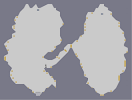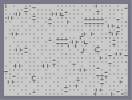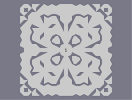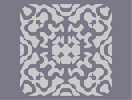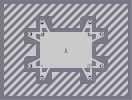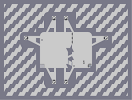Peak 4` Every Last One FLOWER Snowflake Laser Battery US Customs

Pages: (0)

### lol

I got a bunch of gold. But then I died. Lol. Anyways, this looks quite cool. Good job.
Demo Data 8263:0|0|0|0|0|0|17895680|17895697|17895697|17895697|17895697|17895697|89480465|89478485|17895765|17895697|17895697|53547281|35795763|139810|17895697|97587473|89478485|89478485|89478485|89478485|89478485|72701269|68|35782656|35791394|35791394|35791394|35791394|35791394|107376162|107374182|107374182|107374182|107374182|107374182|107374182|107374182|89482854|1118481|35791360|115483170|107374182|107374182|107374182|107374182|107374182|107374182|40265318|35791394|201326594|89474116|89478485|89478485|22369621|17895697|35790848|35782658|33554466|139810|17895696|35782656|53687074|89510161|89478485|89478485|89478485|89478485|89478485|89478485|89478485|89478485|18175317|53687089|35791394|35791394|35791394|35791394|35791394|35791394|107376162|107374182|107374182|107374182|107374182|107374182|107374182|107374182|107374182|89618022|17895701|17895697|17895697|17895697|17895697|17895697|89478493|89478485|89478485|89478485|89478485|89478485|107378517|107374182|107374182|107374182|107374182|107374182|35791394|35791394|35791394|73851426|89408580|89478485|89478485|89478485|89478485|89478485|89478485|107374455|22369621|35790849|71614466|71582788|107374182|107374182|107374182|89478485|89478485|107378517|107374182|107374182|107374182|17896789|71614464|71582788|107234372|107374182|107374182|71591526|89474116|89478485|89478485|89478485|89478485|89478485|33555524|107880994|107374182|71591526|71582788|71582788|71582788|89408580|89408580|89478485|89478485|89478485|89478485|17895701|17895697|17895697|89480465|89478485|89478485|89478485|89478485|89478485|89478485|89478485|89478485|89478485|89478485|219223381|89478485|1118549|0|0|0|79691776|107365444|107374182|71591526|17896789|17895697|17895697|4369|139810|35791394|79692322|71582788|89478212|89478485|89478485|89478485|89478485|123032917|125269879|107374183|107374182|107374182|35791398|71583458|71582788|71582788|72701269|71582788|89474116|72701269|107374180|107374182|107374182|17826886|79692049|71582788|71582788|89474116|125130069|107374183|107374182|107374182|107374182|107374182|91711078|17895765|71587281|71582788|71582788|71582788|107374182|107374182|107374182|107374182|89548390|89478485|22369621|17895697|17895697|89985297|71587157|107373636|107374182|125199974|72701271|105137220|71582790|4473924|17891328|273|237117984|71591526|71582788|71652692|89408580|89478485|89478485|89478485|89478485|107374182|107374182|35808870|115483170|71582788|71582788|89474116|89478485|89478485|89478485|89478485|89478485|89478485|279893|35791392|35791394|107376162|107374182|71582790|71582788|107374180|107374182|107374182|125199974|89478485|89478485|89478485|17895697|71614465|71582788|107234372|71722598|71582788|71582788|88360004|71582789|105137220|107374182|279620|0|17895680|71582813|71582788|107374182|107374182|89478212|89478485|89478485|107304277|35808870|34|79691776|71582788|71582788|79692868|71582788|71582788|73819748|89408580|89478485|89478485|89478485|36070980|35791394|8738|0|71584768|71582788|71582788|71582788|89474116|71652693|71722596|89478484|89408580|107373636|35791462|71583458|71582788|71582788|71582788|89478484|71582788|71582788|71582788|4473924|35791392|35791394|35791394|35791394|35791394|35791394|35791394|35791394|35791394|35791394|35791394|35791394|35791394|35791394|35791394|35791394|35791394|35791394|35791394|35791394|107405858|107374182|40265318|107880994|107374182|107374182|107374182|107374182|35792486|107376162|107374182|107374182|107374182|107374182|107374182|107374182|107374182|35808870|107374306|107374182|107374182|40265318|107405858|107374182|107374182|107374182|115484262|107374182|107374182|107374182|237118054|107374182|107374182|107374182|36071014|107376162|107374182|107374182|107374182|107374182|107880998|107374182|107374182|107374182|107374182|139878|89478476|89478485|89478485|279637|35791394|34|35791392|35791394|35791394|35791394|35791394|35791394|35791394|35791394|35791394|35791394|35791394|107880994|107374182|107374182|35808870|107374306|107374182|107374182|107374182|36071014|107880994|107374182|107374182|34|0|0|35790848|35791394|35791394|88866850|89478485|89478485|89478485|89478485|89478485|89478485|89478485|89478485|89478485|89478485|89478485|89478485|17895701|17895697|17895697|17895697|17895697|17895697|53687089|53687091|53678355|35791411|35791394|35791394|35791394|35791394|35791394|35791394|35791394|35791394|35791394|35791394|35791394|35791394|35791394|35791394|35791394|35791394|35791394|35791394|107405858|107374182|35808870|107405858|107374182|107374182|107374182|107374182|201326626|89478468|89478485|89478485|89478485|17895697|17895697|17895697|35791360|546|17895697|17895697|51450129|35795763|35791394|35791394|35791394|35791394|35791394|35791394|35791394|35791394|35791394|35791394|35791394|35791394|35791394|107376162|35808870|35791394|107374190|107374182|107374182|107374182|36071014|79692322|89478485|89478485|89478485|89478485|89478485|89478485|89478485|89478485|89478485|107444087|107374182|107374182|107374182|107374182|35791398|35791394|35791394|35791394|35791394|35791394|35791394|35791394|35791394|35791394|35791394|107405858|107374182|201335398|89478484|89478485|89478485|89478485|17896789|273|0|0|0|35791394|35791394|35791394|35791394|35791394|35791394|35791394|35791394|35791394|35791394|35791394|115483170|107374182|107374182|140902|89478476|89478485|89478485|22369621|1118481|0|35782656|35791394|35782690|35782690|35791394|35791394|35791394|35791394|35791394|35791394|35791394|35791394|107374190|40265318|88866818|89478485|89478485|89478485|22369621|17895697|273|0|35791392|35791394|35791394|35791394|35791394|35791394|35791394|35791394|35791394|35791394|35791394|107376162|107374182|107374182|79692326|89478212|89478485|89478485|17895765|4369|0|0|35790848|35791394|35791394|35791394|35791394|35791394|35791394|35791394|35791394|35791394|35791394|107376162|157286|89478336|89478485|89478485|89478485|89478485|89478485|89478485|89478485|89478485|89478485|89478485|89478485|89478485|89478485|89478485|17895697|17895697|17895697|17895697|17895697|17895697|89478609|89478485|17913173|107266049|107374182|107374182|107374182|107374182|107374182|107374182|107374182|107374182|107374182|107374182|124151398|20132727|17895697|17895697|17895697|17895697|17895697|17895697|17895697|17895697|17895697|17895697|17895697|17895697|17895697|89480465|89478485|22369621|105168897|107374182|107374182|107374182|107374182|35791462|17891362|35790849|35791394|8738|17895697|17895697|17895697|17895697|17895697|17895697|17895697|17895697|17895697|17895697|17895697|17895697|219222289|89478485|89478485|1118481|107367424|107374182|107374182|107374182|107374182|107374182|107374182|107374182|124151398|89618295|89478485|89478485|89478485|89478485|17896789|17895697|97587473|89478485|89478485|89478485|107374182|107374182|139814|0|0|17895680|17895697|17895697|17895697|17895697|22369745|107266049|107374182|107374182|35791394|35791394|35791394|35791394|20132642|17895697|17895697|17895697|17895697|17895697|17895697|17895697|17895697|17895697|17895697|17895697|17895697|89985297|22369621|1|107373760|107374182|35791394|35791394|35791394|35791394|53687074|17895697|17895697|17895697|17895697|17895697|17895697|17895697|17895697|17895697|17895697|17895697|89480465|70997|107374156|107374182|71582788|107365444|73819750|17895428|17895697|17895697|51450129|35791667|53687074|17895697|17895697|17895697|17895697|17895697|17895697|89985297|17913173|105644033|107374182|107374182|35808870|546|35791360|35791394|35791394|35791394|18035507|17895697|17895697|17895697|17895697|17895697|17895697|17895697|17895697|17895697|17895697|17895697|17895697|97587473|22369621|79692049|107374182|107374182|36071014|35791394|35791394|35791394|35791394|35791394|35791394|20132658|17895697|17895697|17895697|17895697|17895697|17895697|17895697|17895697|17895697|17895697|17895697|17895697|17895697|17895697|22876433|89480465|89478485|17895701|17895697|219222289|105137221|107374182|107374182|107374182|107374182|20145766|35651857|35791394|35791394|53687074|17895731|17895697|17895697|17895697|17895697|17895697|17895697|17895697|17895697|17895697|17895697|17895697|17895697|17895697|18175441|89480465|89478485|89478485|89478485|18175317|107741201|107374182|107374182|35792486|2236962|35790848|34|0|33554432|52568610|17904435|17895697|17895697|17895697|17895697|17895697|17895697|17895697|17895697|17895697|17895697|17895697|17895697|89480465|89478485|89480465|89478485|89478485|89478485|89478485|107266049|107374182|107374182|107374182|35791394|35791394|35791394|35791394|35791394|139810|0|0|17895424|17895697|17895697|17895697|17895697|17895697|17895697|17895697|17895697|17895697|17895697|17895697|17895697|17895697|17895697|17895697|22369629|17895697|89480465|89478485|89478485|89478485|89478485|105644049|107374182|107374182|107374182|107374182|35792486|35791394|35791394|35791394|35791394|35791394|35791394|35791394|53686818|17895731|17895697|17895697|17895697|17895697|17895697|17895697|17895697|17895697|17895697|17895697|17895697|17895697|17895697|17895697|17895697|89510161|97587477|89478485|89478485|89478485|89478485|201330961|107374180|107374182|36071014|34|0|2236962|35790848|35791394|35791394|35791394|35791394|53682722|17895699|17895697|17895697|17895697|17895697|17895697|17895697|17895697|17895697|17895697|17895697|17895697|89480465|22369621|17895697|89478609|89478485|89478485|36909841|53682722|17895697|17895697|89478609|89480465|89478485|89478485|89478485|18175317|105644032|107374182|107374182|107374182|35791398|0|0|35782656|35651586|35791394|35791394|35791394|35791394|18035507|17895697|17895697|17895697|17895697|17895697|17895697|17895697|17895697|17895697|17895697|17895697|17895697|17895697|17895697|17895697|17913181|97587473|89478485|89478485|89478485|89478485|1135957|107373760|107374182|107374182|107374182|107374182|107374182|107374182|107374182|107374182|107374182|107374182|107374182|89618295|17895701|17895697|17895697|17895697|17895697|17895697|17895697|17895697|17895697|17895697|17895697|17895697|17895697|17895697|17895697|219222289|89478485|89478485|97588565|89478485|89478485|89478485|89478485|89478485|72089617|107374182|107374182|107374182|107374182|107374182|107374182|107374182|107374182|107374182|107374182|107374182|107374182|89618278|89478485|89478485|89478485|17913173|17895697|17895697|17895697|17895697|17895697|17895697|17895697|17895697|17895697|17895697|219222289|89478485|89478485|89478485|279621|89510161|89478485|89478485|89478485|22369621|89510161|89478485|89478485|89478485|89478485|105644100|107374182|107374182|107374182|35791462|35791394|35791394|546|0|17825792|17895697|17895697|17895697|17895697|17895697|273

### i would

give this a 3 but you must have took forever on this so 4

### lol

with all the lag of the real thing!

### wow

that thumbnail looks awsome

### Oh... My... God...

LOL that must have taken a loooong time!

### lol

ALL GOLD DEMO XDDD
Demo Data 4309:0|0|0|17825792|17895697|17895697|17895697|17895697|17895697|89480465|89478485|89478485|89478485|89478485|89478485|89478485|89478485|89478485|89478485|89478485|17896789|89510161|89478485|89478485|89478485|17913173|79691777|107234372|107374182|107374182|107374182|35791462|35791394|35791394|35791394|35791394|35791394|35791394|35791394|35791394|107880994|107374182|107374182|36071014|115483170|107374182|107374182|107374182|107374182|35791398|35791394|34|17891328|17895697|17895697|17895697|17895697|17895697|89510161|89478485|89478485|89478485|89478485|89478485|89478485|89478485|89478485|89478485|18175317|17895697|89478493|17896789|219222289|89478485|89478485|89478485|89478485|1118549|72089600|33554500|2236962|0|79691776|71582788|1092|17895697|17895697|89478493|18175317|72732945|71582788|107374148|35791462|35791394|35791394|35791394|35791394|35791394|35791394|35791394|35791394|35791394|35791394|35791394|107405858|107374182|107374182|107374182|35792486|35791394|35791394|35791394|35791394|546|17895424|17895697|17895697|17895697|17895697|89985297|89478485|17896789|17895697|17895697|17895697|17895697|17895697|17895697|17895697|89510161|89478485|89478485|89478485|89478485|89478485|89478485|89478485|89478485|89478485|71652693|17476|35791392|107374190|107374182|107374182|107374182|107374182|35791394|35791394|35791394|35791394|35791394|35791394|35791394|107374190|36071014|35791394|35791394|35791394|35791394|35791394|35791394|35791394|107374306|107374182|107374182|107374182|36071014|35791394|72089600|89478212|17895765|4369|71582796|89478212|89478485|89478485|89478485|89478485|89478485|89478485|89478485|89478485|89478485|89478485|89478485|89478485|89478485|89478485|89478485|89478485|89478485|89478485|89478485|89478485|89478485|89478485|89478485|17895697|219223389|4474197|35791360|35791360|2|35790848|35791394|34|0|0|16777216|17895697|17895697|89478609|71587157|71582788|71582788|71582788|71582788|35790848|35791394|35791394|35791394|139810|17600|71582912|71582788|71582788|71582788|0|0|71582796|71582788|71582788|71582788|71582788|89478212|89478485|89478485|17895701|17895697|1|72089600|107365444|107374182|107374182|107374182|107374182|107374182|107374182|107374182|107374182|35791394|35791394|35791394|35791394|35791394|35791394|35791394|35791394|35791394|35791394|71722722|71582788|71582788|71582788|4473924|0|0|0|0|0|0|0|0|71582912|71582788|71582788|71582788|201326596|71582788|71582788|89478484|89478485|89478485|89478485|89478485|89478485|89478485|89478485|17895697|17895697|17895697|17895697|89478609|72701269|71582788|71582788|71582788|71582788|71582788|71582788|71582788|71582788|16777284|17895697|97587473|89478485|89478485|89478485|1118549|71614464|71582788|71582788|105137220|36071014|35791394|35791394|35791394|35791394|35791394|35791394|35791394|35791394|35791394|71591534|71582788|71582788|71582788|71582788|71582788|71582788|71582788|71582788|1092|35791394|35791394|35791394|107405858|107374182|107374182|107374182|35808870|35791394|115483170|139878|89476096|89478485|89478485|1119573|35790848|35791394|35791394|35791394|107880994|107374182|107374182|107374182|201344070|71582788|71582788|71582788|89478485|89478485|89478485|89478485|89478485|17896789|17895697|17895697|17895697|17895697|17895697|17895697|89480465|89478485|89478485|89478485|89478485|89478485|89478485|89478485|89478485|89478485|17913173|89478609|89478485|89478485|89478485|89478485|89478485|1118549|72089600|71582788|17476|0|35791394|35791394|35791394|35791394|2236962|17895680|17895697|17895697|17895697|17895697|17895697|17895697|89510161|71587157|1092|71584768|71582788|71582788|71582788|4473924|0|17891328|1118481|35651584|35791394|35791394|546|17895680|17895697|17895697|17895697|17895697|89478609|89478485|89478485|219223381|89478485|89478485|89478485|89478485|89478485|69973|71582912|71582788|71582788|107374182|35791398|35791394|35791394|35791394|35791394|35791394|35791394|35791394|35791394|35791394|35791394|35791394|237117986|107374182|107374182|107374182|107374182|107374182|107374182|107374182|107374182|107374182|107374182|35792486|139810|16777216|17895697|273|35782656|115483170|107374182|107374182|107374182|35792486|115483170|107374182|107374182|107374182|107374182|107374182|107374182|35792486|8738|79691776|71582788|35782660|35791394|35791394|35791394|35791394|35791394|35791394|139810|17895680|273|0|35791394|35791394|115483170|107374182|107374182|35792486|107374190|107374182|107374182|107374182|40265318|71724578|88360004|89478485|89478485|89478485|89478485|89478485|89478485|89478485|17913173|17895697|17895697|17895697|17895697|17895697|17895697|17895697|17895697|17895697|17895697|17895697|17895697|17895697|89480465|89478485|18175317|89480465|89478485|89478485|89478485|89478485|17895697|89478609|71582805|107373636|107374182|107374182|107374182|107374182|36071014|35791394|35791394|35791394|35791394|35791394|35791394|35791394|35791394|35791394|35791394|35791394|35791394|35791394|35791394|35791394|35791394|35791394|115483170|107374182|107374182|40265318|35791394|107374190|107374182|107374182|107374182|107374182|40265318|115483170|107374182|71582788|71582788|68|35790848|35791394|35791394|35791394|237117986|107374182|107374182|107374182|107374182|107374182|107405858|107374182|107374182|107374182|107880994|107374182|107374182|107374182|107374182|2238054|71584768|89478212|89478485|89478485|89478485|89478485|89478485|17895701|17895697|17895697|17895697|17895697|17895697|17895697|69905